# Texas Go Math Grade 5 Lesson 5.2 Answer Key Subtraction with Unequal Denominators

Refer to our Texas Go Math Grade 5 Answer Key Pdf to score good marks in the exams. Test yourself by practicing the problems from Texas Go Math Grade 5 Lesson 5.2 Answer Key Subtraction with Unequal Denominators.

## Texas Go Math Grade 5 Lesson 5.2 Answer Key Subtraction with Unequal Denominators

Investigate

Mario fills a hummingbird feeder with $$\frac{3}{4}$$ cup of sugar water on Friday. On Monday, Mario sees that $$\frac{1}{8}$$ cup of sugar water is left. How much sugar water did the hummingbirds drink?

Materials: fraction strips; MathBoard

A. Find $$\frac{3}{4}$$ – $$\frac{1}{8}$$. Place three $$\frac{1}{4}$$ strips under the 1-whole strip on your MathBoard. Then place a $$\frac{1}{8}$$ strip under the $$\frac{1}{4}$$ strips.B. Find fraction strips all with the same denominator that fit exactly under the difference $$\frac{3}{4}$$ – $$\frac{1}{8}$$.C. Record the difference, $$\frac{3}{4}$$ – $$\frac{1}{8}$$ = ___________

So, the hummingbirds drank _________ cup of sugar water.

C. Record the difference, $$\frac{3}{4}$$ – $$\frac{1}{8}$$ = $$\frac{6}{8}$$ – $$\frac{1}{8}$$ = $$\frac{5}{8}$$

So, the hummingbirds drank $$\frac{5}{8}$$ cup of sugar water.

Math Talk
Mathematical Processes

How can you tell if the difference of the fractions is less than 1? Explain.
Answer: $$\frac{5}{8}$$ is less than one
by using the fraction strip method
Explanation: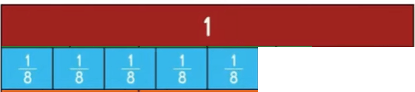Draw Conclusions

Question 1.
Describe how you determined what fraction strips, all with the same denominator, would fit exactly under the difference. What are they?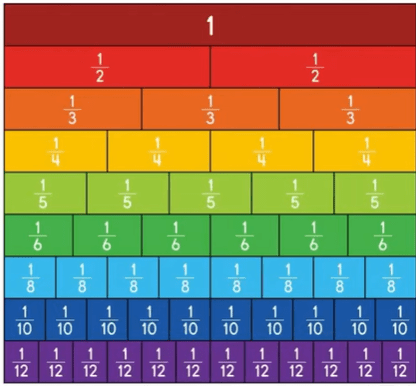Explanations:
Fraction strips, all with the same denominator, would fit exactly under the difference.
the above figure explains

Question 2.
H.O.T. Explain whether you could have used fraction strips with any other denominator to find the difference. If so, what is the denominator?
Answer: Un equal denominator
Explanation:
used fraction strips with any other denominator to find the difference. If so, that is called unequal denominator

Make Connections

Sometimes you can use different sets of same-denominator fraction strips to find the difference. All of the answers will be correct.

Solve. $$\frac{2}{3}$$ – $$\frac{1}{6}$$

A. Find fraction strips, all with the same denominator, that fit exactly under the difference $$\frac{2}{3}$$ – $$\frac{1}{6}$$.$$\frac{2}{3}$$ – $$\frac{1}{6}$$ = $$\frac{3}{6}$$

B. Find another set of fraction strips, all with the same denominator, that fit exactly under the difference $$\frac{2}{3}$$ – $$\frac{1}{6}$$. Draw the fraction strips you used.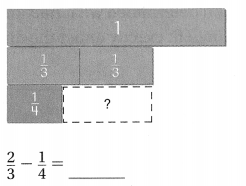$$\frac{2}{3}$$ – $$\frac{1}{6}$$ = ___________

C. Find other fraction strips, all with the same denominator, that fit exactly under the difference $$\frac{2}{3}$$ – $$\frac{1}{6}$$. Draw the fraction strips you used.
$$\frac{2}{3}$$ – $$\frac{1}{6}$$ = ____________

While each answer appears different, all of the answers can be simplified to _________.

A. Find fraction strips, all with the same denominator, that fit exactly under the difference $$\frac{2}{3}$$ – $$\frac{1}{6}$$.$$\frac{2}{3}$$ – $$\frac{1}{6}$$ = $$\frac{3}{6}$$
$$\frac{7}{10}$$ – $$\frac{2}{5}$$ =$$\frac{7}{10}$$ – $$\frac{4}{10}$$ = $$\frac{3}{10}$$

B. Find another set of fraction strips, all with the same denominator, that fit exactly under the difference $$\frac{2}{3}$$ – $$\frac{1}{6}$$. Draw the fraction strips you used.$$\frac{2}{3}$$ – $$\frac{1}{6}$$ =$$\frac{3}{6}$$
$$\frac{2}{3}$$ – $$\frac{1}{4}$$ =$$\frac{8}{12}$$ – $$\frac{3}{12}$$ = $$\frac{5}{12}$$

C. Find other fraction strips, all with the same denominator, that fit exactly under the difference $$\frac{2}{3}$$ – $$\frac{1}{6}$$. Draw the fraction strips you used.
$$\frac{2}{3}$$ – $$\frac{1}{6}$$ =$$\frac{3}{6}$$While each answer appears different, all of the answers can be simplified to equal denominators

Math Talk
Mathematical Processes

Which other fraction strips with the same denominator could fit exactly in the difference of $$\frac{2}{3}$$ – $$\frac{1}{6}$$?
Answer: $$\frac{3}{6}$$
Explanation: fraction strips with the same denominator could fit exactly in the difference of $$\frac{2}{3}$$ – $$\frac{1}{6}$$ is $$\frac{3}{6}$$

Share and Show

Use fraction strips to find the difference. Write your answer in the simplest form.

Question 1.$$\frac{7}{10}$$ – $$\frac{2}{5}$$ =$$\frac{7}{10}$$ – $$\frac{4}{10}$$ = $$\frac{3}{10}$$
Explanation:
The fraction with unequal denominators are subtracted to get the sum
By doing them to equal denominators

Question 2.$$\frac{2}{3}$$ – $$\frac{1}{4}$$ =$$\frac{8}{12}$$ – $$\frac{3}{12}$$ = $$\frac{5}{12}$$
Explanation:
The fraction with unequal denominators are subtracted to get the sum
By doing them to equal denominators

Use fraction strips to find the difference. Write your answer in simplest form.

Question 3.
$$\frac{3}{4}$$ – $$\frac{1}{3}$$ = _____________
$$\frac{3}{4}$$ – $$\frac{1}{3}$$ =$$\frac{9}{12}$$ – $$\frac{4}{12}$$ = $$\frac{5}{12}$$
Explanation:
The fraction with unequal denominators are subtracted to get the sum
By doing them to equal denominators

Question 4.
$$\frac{5}{6}$$ – $$\frac{1}{2}$$ = ______________
$$\frac{5}{6}$$ – $$\frac{1}{2}$$ = $$\frac{5}{6}$$ – $$\frac{3}{6}$$= $$\frac{2}{6}$$
Explanation:
The fraction with unequal denominators are subtracted to get the sum
By doing them to equal denominators

Question 5.
$$\frac{3}{4}$$ – $$\frac{7}{12}$$ = ______________
$$\frac{3}{4}$$ – $$\frac{7}{12}$$ = $$\frac{9}{12}$$ – $$\frac{7}{12}$$ =
$$\frac{2}{12}$$
Explanation:
The fraction with unequal denominators are subtracted to get the sum
By doing them to equal denominators

Unlock the Problem

Question 6.
H.O.T. Multi-Step The picture at the right shows how much pizza was left over from lunch. Jason eats $$\frac{1}{4}$$ of the whole pizza for dinner. Which subtraction sentence represents the amount of pizza that is remaining after dinner?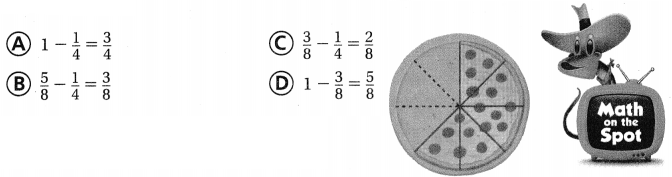a. What problem are you being asked to solve?
Answer: fractions with unequal denominators

b. How will you use the diagram to solve the problem?
By calculating, Number of slices ate by number of slices are not eaten

c. Jason eats 1 of the whole pizza. How many slices does he eat?
Answer: $$\frac{1}{8}$$

d. Redraw the diagram of the pizza. Shade the sections of pizza that are remaining after Jason eats his dinner.e. Write a fraction to represent the amount of pizza that is remaining.
Answer: $$\frac{5}{8}$$

f. Fill in the bubble for the correct answer choice above.

Question 7.
H.O.T. Explain how a model for $$\frac{3}{5}$$ – $$\frac{1}{2}$$ is different from a model for $$\frac{3}{5}$$ – $$\frac{3}{10}$$.
$$\frac{3}{5}$$ – $$\frac{1}{2}$$ = $$\frac{6}{10}$$ – $$\frac{5}{10}$$ = $$\frac{1}{10}$$
$$\frac{3}{5}$$ – $$\frac{3}{10}$$ = $$\frac{6}{10}$$ – $$\frac{3}{10}$$ =
$$\frac{3}{10}$$
They both are same
subtraction with unequal denominator
both get the denominator same
with numerator change

Fill in the bubble completely to show your answer.

Question 8.
You are making cranberry lemonade for the Tastiest Beverage contest. You use $$\frac{3}{10}$$ liter cranberry juice and $$\frac{1}{2}$$ liter lemonade. You drink $$\frac{1}{10}$$ liter, just to be sure that it tastes delicious! How much cranberry lemonade do you have left?
(A) $$\frac{7}{10}$$ liter
(B) $$\frac{9}{10}$$ liter
(C) $$\frac{3}{11}$$ liter
(D) $$\frac{3}{10}$$ liter
$$\frac{3}{10}$$ + $$\frac{1}{2}$$ = $$\frac{3}{10}$$ + $$\frac{5}{10}$$ = $$\frac{8}{10}$$
$$\frac{8}{10}$$ – $$\frac{1}{10}$$ =$$\frac{7}{10}$$

Question 9.
Use Diagrams Calvin used fraction strips correctly to model the difference of $$\frac{7}{12}$$ – $$\frac{1}{3}$$. Which of these describes his model?
(A) seven $$\frac{1}{12}$$ strips, one $$\frac{1}{3}$$ strip, two $$\frac{1}{4}$$ strips
(B) seven $$\frac{1}{12}$$ strips, one $$\frac{1}{3}$$ strip, one $$\frac{1}{2}$$ strip
(C) seven $$\frac{1}{12}$$ strips, two $$\frac{1}{6}$$ strips, one $$\frac{1}{8}$$ strip
(D) seven $$\frac{1}{12}$$ strips, one $$\frac{1}{3}$$ strip, one $$\frac{1}{4}$$ strip

Question 10.
Multi-Step Bethany made her Apple Surprise drink by mixing $$\frac{1}{8}$$ pint lemon juice, $$\frac{1}{8}$$ pint grape juice, and $$\frac{4}{8}$$ pint apple juice. She then drank $$\frac{1}{4}$$ pint of the mixture. How much Apple Surprise was left?
(A) $$\frac{1}{2}$$ pint
(B) $$\frac{1}{8}$$ pint
(C) $$\frac{1}{4}$$ pint
(D) $$\frac{3}{8}$$ pint
$$\frac{1}{8}$$ + $$\frac{1}{8}$$ + $$\frac{4}{8}$$ = $$\frac{6}{8}$$
$$\frac{6}{8}$$ – $$\frac{1}{4}$$ = $$\frac{6}{8}$$ – $$\frac{2}{8}$$ = $$\frac{1}{2}$$

Texas Test Prep

Question 11.
The diagram shows what Tina had left from a yard of fabric. She now uses a yard of fabric for a project. How much of the original yard of fabric
does Tina have left after the project?(A) $$\frac{1}{2}$$ yard
(B) $$\frac{2}{3}$$ yard
(C) $$\frac{1}{3}$$ yard
(D) $$\frac{1}{6}$$ yard
Tina had left from a yard of fabric. She now uses a yard of fabric for a project. $$\frac{5}{6}$$ yard
$$\frac{1}{6}$$ yard is left

### Texas Go Math Grade 5 Lesson 5.2 Homework and Practice Answer Key

Use fraction strips to find the difference. Write your answer in the simplest form.

Question 1.$$\frac{7}{8}$$ – $$\frac{1}{4}$$ = $$\frac{7}{8}$$ – $$\frac{2}{8}$$ =
$$\frac{5}{8}$$
Explanation:
The fraction with unequal denominators are subtracted to get the sum
By doing them to equal denominators

Question 2.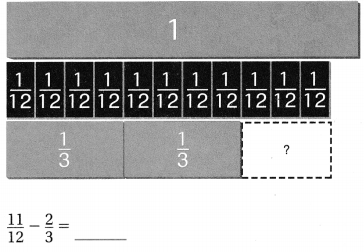$$\frac{11}{12}$$ – $$\frac{2}{3}$$ = $$\frac{11}{12}$$ – $$\frac{8}{12}$$ =
$$\frac{3}{12}$$
Explanation:
The fraction with unequal denominators are subtracted to get the sum
By doing them to equal denominators

Question 3.
$$\frac{1}{2}$$ – $$\frac{1}{3}$$ = _____________
$$\frac{1}{2}$$ – $$\frac{1}{3}$$ = $$\frac{3}{6}$$ – $$\frac{2}{6}$$ =
$$\frac{1}{6}$$
Explanation:
The fraction with unequal denominators are subtracted to get the sum
By doing them to equal denominators

Question 4.
$$\frac{9}{10}$$ – $$\frac{2}{5}$$ = _____________
$$\frac{9}{10}$$ – $$\frac{2}{5}$$ = $$\frac{9}{10}$$ – $$\frac{4}{10}$$ = $$\frac{5}{10}$$
Explanation:
The fraction with unequal denominators are subtracted to get the sum
By doing them to equal denominators

Question 5.
$$\frac{11}{12}$$ – $$\frac{3}{4}$$ = _____________
$$\frac{11}{12}$$ – $$\frac{3}{4}$$ =  $$\frac{11}{12}$$ – $$\frac{9}{12}$$ =$$\frac{1}{6}$$
Explanation:
The fraction with unequal denominators are subtracted to get the sum
By doing them to equal denominators

Question 6.
$$\frac{5}{6}$$ – $$\frac{1}{3}$$ = _____________
Explanation:
The fraction with unequal denominators are subtracted to get the sum
By doing them to equal denominators

Question 7.
$$\frac{2}{3}$$ – $$\frac{1}{12}$$ = _____________
Explanation:
The fraction with unequal denominators are subtracted to get the sum
By doing them to equal denominators

Question 8.
$$\frac{3}{4}$$ – $$\frac{5}{12}$$ = _____________
Explanation:
The fraction with unequal denominators are subtracted to get the sum
By doing them to equal denominators

Question 9.
$$\frac{9}{10}$$ – $$\frac{1}{2}$$ = _____________
Explanation:
The fraction with unequal denominators are subtracted to get the sum
By doing them to equal denominators

Question 10.
$$\frac{5}{8}$$ – $$\frac{1}{2}$$ = _____________
$$\frac{5}{8}$$ – $$\frac{1}{2}$$ = $$\frac{5}{8}$$ – $$\frac{4}{8}$$ = $$\frac{1}{8}$$
Explanation:
The fraction with unequal denominators are subtracted to get the sum
By doing them to equal denominators

Question 11.
$$\frac{3}{4}$$ – $$\frac{2}{3}$$ = _____________
$$\frac{3}{4}$$ – $$\frac{2}{3}$$ = $$\frac{9}{12}$$ – $$\frac{8}{12}$$ = $$\frac{1}{12}$$
Explanation:
The fraction with unequal denominators are subtracted to get the sum
By doing them to equal denominators

Problem Solving

Question 12.
Annette is making a fruit drink that calls for $$\frac{3}{4}$$ cup of fresh lemon juice. She has $$\frac{1}{2}$$ cup of lemon juice. How much more lemon juice does Annette need?
$$\frac{3}{4}$$ – $$\frac{1}{2}$$ = $$\frac{3}{4}$$–$$\frac{2}{4}$$ =  $$\frac{1}{4}$$
Explanation:
The fraction with unequal denominators are subtracted to get the sum
By doing them to equal denominators

Question 13.
Ramon needs to walk $$\frac{3}{4}$$ mile to the bus stop. He has walked $$\frac{3}{8}$$ mile so far. How much farther does Ramon need to walk to get to the bus stop?
$$\frac{3}{4}$$ – $$\frac{3}{8}$$ = $$\frac{6}{8}$$ – $$\frac{3}{8}$$ =$$\frac{3}{8}$$
Explanation:
The fraction with unequal denominators are subtracted to get the sum
By doing them to equal denominators

Lesson Check

Fill In the bubble completely to show your answer.

Question 14.
Matt spent $$\frac{1}{3}$$ of the money in his pocket on a movie ticket. He spent $$\frac{1}{4}$$ of the money on a snack. What fraction of his money is left?
(A) $$\frac{7}{12}$$
(B) $$\frac{5}{12}$$
(C) $$\frac{1}{12}$$
(D) $$\frac{1}{6}$$
Explanation:
$$\frac{1}{3}$$ + $$\frac{1}{4}$$ = $$\frac{4}{12}$$ + $$\frac{3}{12}$$ = $$\frac{7}{12}$$
1 – $$\frac{7}{12}$$ = $$\frac{5}{12}$$
Matt spent $$\frac{1}{3}$$ of the money in his pocket on a movie ticket.
He spent $$\frac{1}{4}$$ of the money on a snack.
$$\frac{5}{12}$$ fraction of his money is left.

Question 15.
Jabar used fraction strips to model the difference of $$\frac{7}{12}$$ –$$\frac{1}{6}$$ Which represents the difference?
(A) seven $$\frac{1}{12}$$ strips
(B) one $$\frac{1}{12}$$ strip
(C) two $$\frac{1}{12}$$ strips
(D) five $$\frac{1}{12}$$ strips
$$\frac{7}{12}$$ – $$\frac{1}{6}$$ = $$\frac{7}{12}$$ – $$\frac{2}{12}$$ = $$\frac{5}{12}$$
Explanation:
Jabar used fraction strips to model the difference of $$\frac{7}{12}$$ –$$\frac{1}{6}$$  represents the difference is five $$\frac{1}{12}$$ strips

Question 16.
Which fraction correctly completes the equation?
$$\frac{3}{4}$$ – _________ = $$\frac{1}{8}$$
(A) $$\frac{7}{8}$$
(B) $$\frac{1}{2}$$
(C) $$\frac{5}{8}$$
(D) $$\frac{1}{4}$$
Explanation:
$$\frac{3}{4}$$ – $$\frac{5}{8}$$ = $$\frac{1}{8}$$

Question 17.
Three friends share a pizza divided into eighths. If each person eats one slice, how many more slices must be eaten so that $$\frac{1}{2}$$ of the pizza remains?
(A) 1
(B) 2
(C) 3
(D) 4
Explanation:
Three friends share a pizza divided into eighths.$$\frac{3}{8}$$
half of the pizza means 4 pieces
If each person eats one slice, 1 more slices must be eaten so that $$\frac{1}{2}$$ of the pizza remains

Question 18.
Multi-Step Sara and Jon each ordered a medium pizza. Sara ate $$\frac{3}{8}$$ of her pizza for lunch and $$\frac{1}{4}$$ for a snack. Jon ate $$\frac{1}{2}$$ of his pizza for lunch and $$\frac{1}{4}$$ for a snack. How much more pizza did Jon eat?
(A) $$\frac{1}{8}$$
(B) $$\frac{1}{4}$$
(C) $$\frac{1}{2}$$
(D) $$\frac{1}{3}$$
Explanation:
Sara and Jon each ordered a medium pizza.
Sara ate $$\frac{3}{8}$$ of her pizza for lunch and $$\frac{1}{4}$$ for a snack.
$$\frac{3}{8}$$ + $$\frac{1}{4}$$ = $$\frac{5}{8}$$
Jon ate $$\frac{1}{2}$$ of his pizza for lunch and $$\frac{1}{4}$$ for a snack.
$$\frac{1}{2}$$ + $$\frac{1}{4}$$ = $$\frac{3}{4}$$
$$\frac{5}{8}$$ – $$\frac{3}{4}$$ = $$\frac{1}{8}$$
Jon eat $$\frac{1}{8}$$

Question 19.
Multi-Step On field day, $$\frac{1}{10}$$ of the students in Mrs. Brown’s class competed in jumping events, $$\frac{3}{5}$$ of the students competed in running events, and $$\frac{1}{10}$$ competed in throwing events. What part of Mrs. Brown’s class did not compete in jumping, running, or throwing events?
(A) $$\frac{1}{5}$$
(B) $$\frac{7}{10}$$
(C) $$\frac{2}{5}$$
(D) $$\frac{4}{5}$$
On field day, $$\frac{1}{10}$$ of the students in Mrs. Brown’s class competed in jumping events,
$$\frac{3}{5}$$ of the students competed in running events,
and $$\frac{1}{10}$$ competed in throwing events.
$$\frac{1}{10}$$ + $$\frac{3}{5}$$ + $$\frac{1}{10}$$ = $$\frac{1}{10}$$ + $$\frac{6}{10}$$ + $$\frac{1}{10}$$ = $$\frac{8}{10}$$ – 1= $$\frac{1}{5}$$